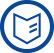### Mission

The project-oriented research at the Weierstrass Institute is characterized by combining the mathematical disciplines of analysis, stochastics and numerics. This combination has great potential for solving complex applied problems such as the reliable extraction of information from large datasets or the suitable consideration of uncertainties in describing processes. In this way, the institute aids in solving current societal challenges.

more

### Research

The institute dedicates itself to fundamental mathematical research as well as the development of algorithms and scientific software. During the problem-solving process, mathematical models of physical and technological systems are designed that properly capture observed phenomena, thereby providing access to highly developed mathematical analysis. At WIAS the phases of the solving process are repeated and coordinated until an optimal solution is found.

more

## Mathematical Core Areas

The WIAS is organised in eight Research Groups specializing in different mathematical techniques and methods. Additional temporary teams are created within a Flexible Research Platform as the need arises. The core areas of mathematical research are the analysis and numerics of partial differential equations, and stochastics.

more

## Main Areas of Application

Research at WIAS focuses on the following application areas

more

## Cooperation and Consulting

Modern applied mathematical methods are a fundamental resource and driving force for technological and economic development around the world. The rapid development of computer technology and mathematically based numerical methods are making it possible to numerically simulate ever more complex engineering, medical, economic and environmental problems.

At the WIAS excellent fundamental research is combined with years of experience in cooperating successfully with partners in the widest possible mix of application fields. The institute is therefore a recognized expert in the solution of complex economic, scientific, and technical problems by means of mathematical modeling and numerical simulation.

more### News

Schrödinger Fellow Michael Kniely at the WIAS
Mathematician from Graz conducts basic mathematical research for technical solutions.

more

Events
Wednesday, 22.03.2023, 10.00 (WIAS-406)
Joint Research Seminar on Nonsmooth Variational Problems and Operator Equations / Mathematical Optimization
Dr. C. Christof, Technische Universität München:
On the identification and optimization of nonsmooth superposition operators in semilinear elliptic PDEs

further events### Jobs

PhD student position (f/m/d) (22/27)
Multicriteria Optimization Subject to Equilibrium Constraints Using the Example of Gas Networks

PhD student position (f/m/d) (22/29)
Stochastic gradient methods for almost sure state constraints for optimal control of gas flow under uncertainty

Research Assistant Position (f/m/d) (22/40)
Optimization with partial differential equations and variational inequalities

Research Assistant Position (f/m/d) (23/02)
Development, analysis and implementation of discretization methods and solution algorithms for the transport of charged species

Research Associate (f/m/d) (23/04)
Development of infrastructures for mathematics, implementing the FAIR principles and supporting Open Science with focus on statistics and machine learning

Research Associate (f/m/d) (23/05)
Mathematical research data in image processing and biomedical sciences